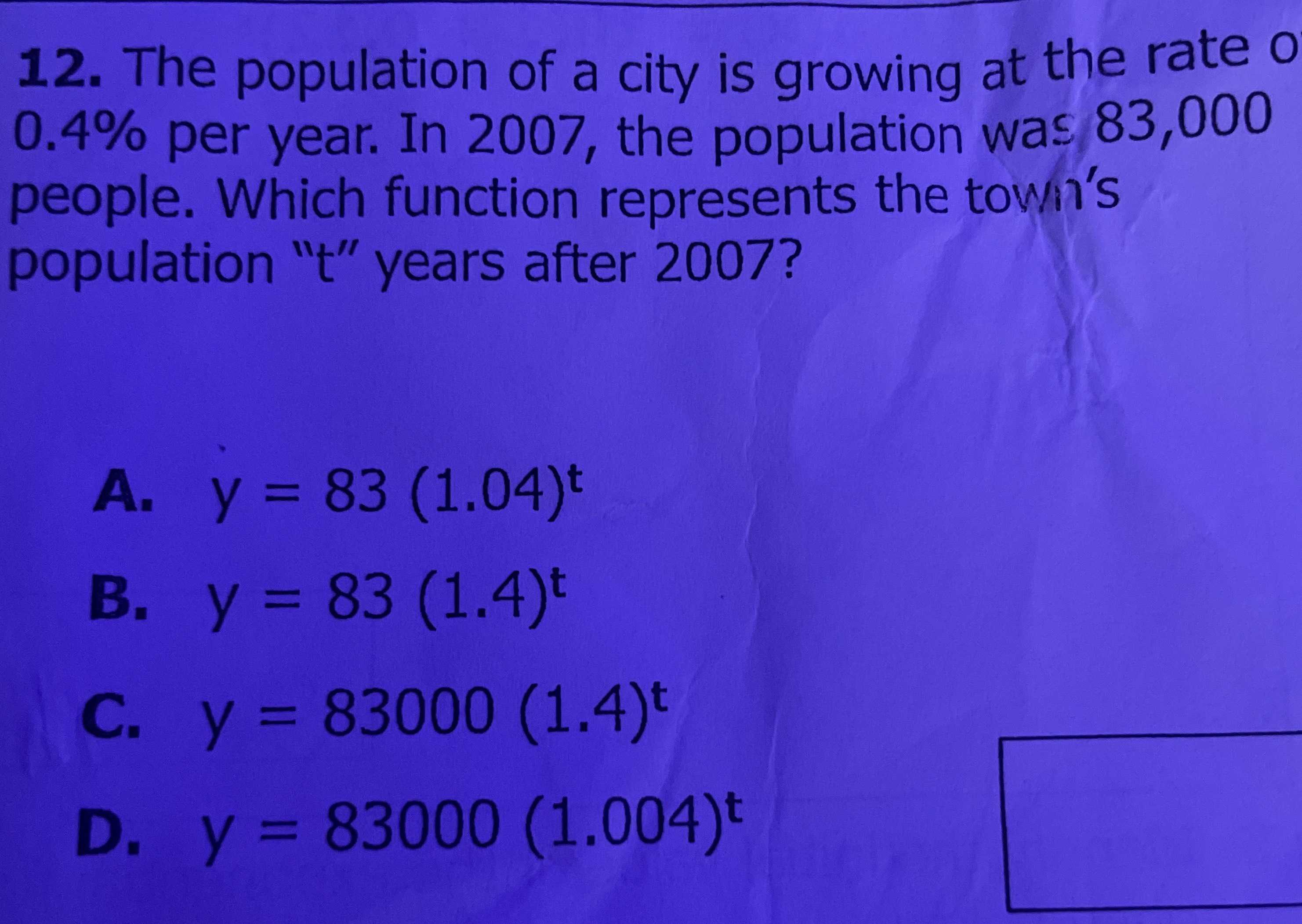### ¿Todavía tienes preguntas de matemáticas?

Pregunte a nuestros tutores expertos
Algebra
Pregunta12. The population of a city is growing at the rate o $$0.4 \%$$ per year. In $$2007$$ , the population was $$83,000$$ people. Which function represents the towhin's population "t" years after $$2007$$ ?

A. $$y = 83 ( 1.04 ) ^ { t }$$

B. $$y = 83 ( 1.4 ) ^ { t }$$

C. $$y = 83000 ( 1.4 ) ^ { t }$$

D. $$y = 83000 ( 1.004 ) ^ { t }$$

y = 83000(1.4 )$$^t$$